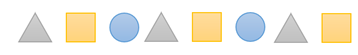Mathematics
Easy

Question

# Select the true statement/s for the repeating pattern. The rule is "Triangle, Square, Circle”:## The next shape is a circle    The 15th shape in the pattern is a circle    The triangle is the 1st, 4th, 7th, etc., shape    All the aboveHint:

## The correct answer is: All the above

### The given pattern in words is Triangle, Square, Circle, Triangle, Square, Circle, Triangle, SquareThere are 8 shapes in the above sequence.Rule : "Triangle, Square, Circle."Let’s check the options one by one.Option A : The next shape is circle.The last shape is Square. According to the rule, the shape after square should be circle. Next shape will be circle.So, the option is right.Option B : The 15th shape in the pattern is circle.The third shape of the rule is circle. And the number of shapes repeating are 3. So, every multiple of 3 will be circle.3 × 5 = 1515 is a multiple of 3. So, 15th shape is circle.This option is right.Option C : The triangle is 1st ,4th, 7th etc., shape.From the figure, we can see triangle is at 1st , 4th, 7th etc., places.This option is right.Option D : All of the above.As all the three options are right, we have to choose the option D.

We have to be careful about the order of the shapes.

### Related Questions to study#### With Turito Foundation.#### Get an Expert Advice From Turito.